Скачать презентацию Chapter 7 Bonds and Their Valuation Chapter

269accf61f4383138915f6c95aaccb8d.ppt

• Количество слайдов: 44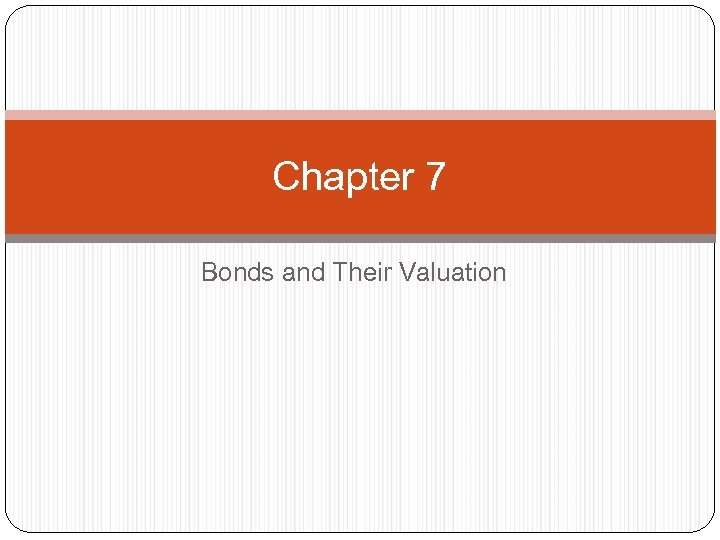Chapter 7 Bonds and Their Valuation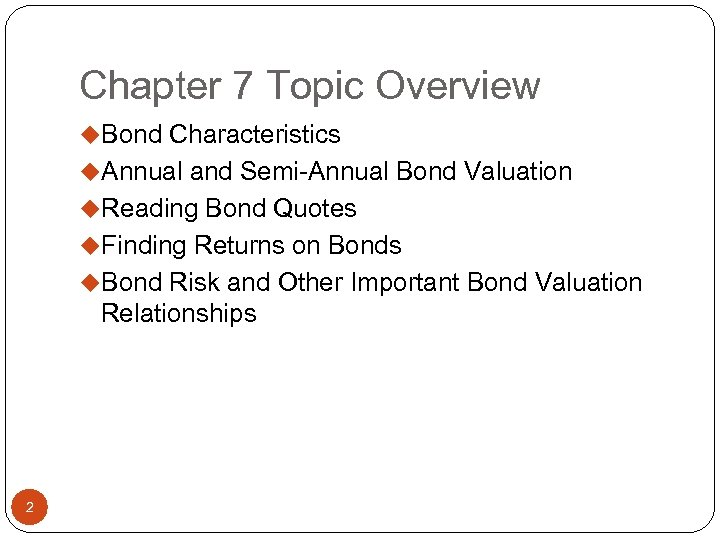Chapter 7 Topic Overview u. Bond Characteristics u. Annual and Semi-Annual Bond Valuation u. Reading Bond Quotes u. Finding Returns on Bonds u. Bond Risk and Other Important Bond Valuation Relationships 2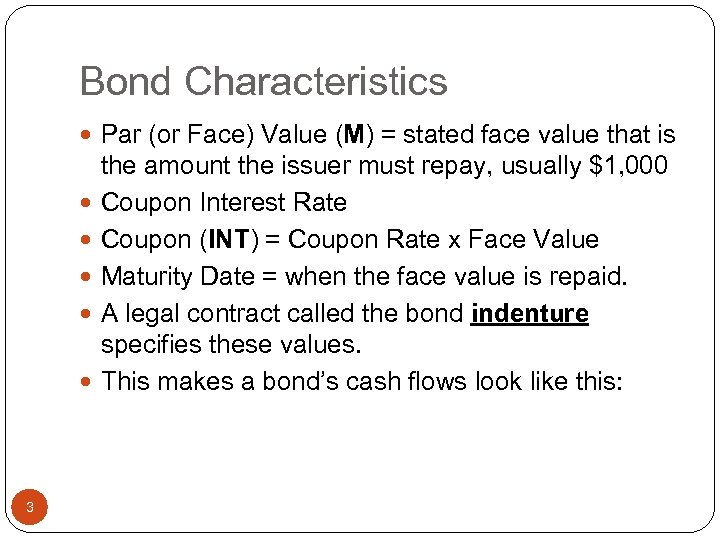Bond Characteristics Par (or Face) Value (M) = stated face value that is 3 the amount the issuer must repay, usually \$1, 000 Coupon Interest Rate Coupon (INT) = Coupon Rate x Face Value Maturity Date = when the face value is repaid. A legal contract called the bond indenture specifies these values. This makes a bond’s cash flows look like this: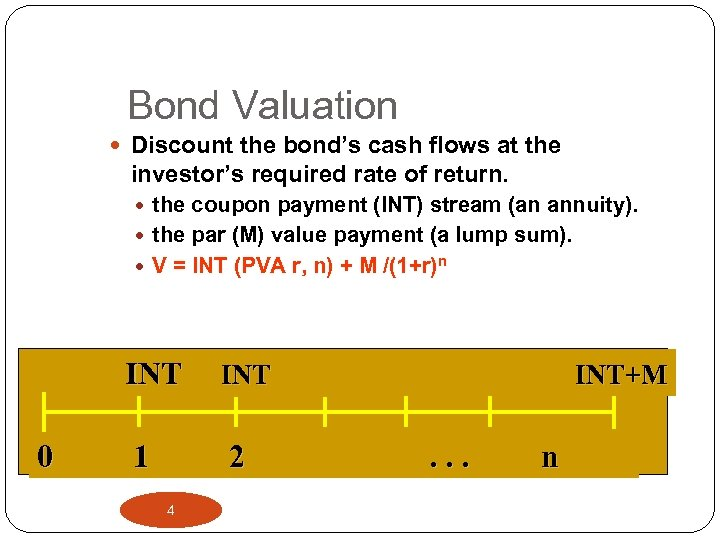Bond Valuation Discount the bond’s cash flows at the investor’s required rate of return. the coupon payment (INT) stream (an annuity). the par (M) value payment (a lump sum). V = INT (PVA r, n) + M /(1+r)n INT 0 INT 1 2 4 INT+M . . . n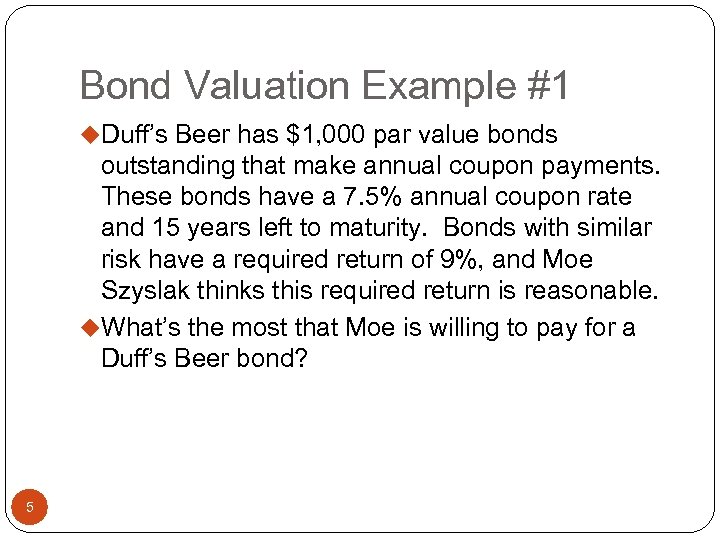Bond Valuation Example #1 u. Duff’s Beer has \$1, 000 par value bonds outstanding that make annual coupon payments. These bonds have a 7. 5% annual coupon rate and 15 years left to maturity. Bonds with similar risk have a required return of 9%, and Moe Szyslak thinks this required return is reasonable. u. What’s the most that Moe is willing to pay for a Duff’s Beer bond? 5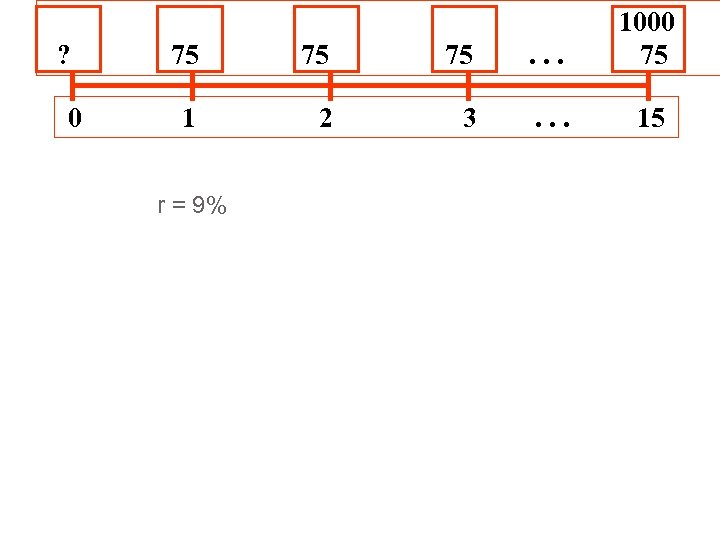? 0 75 75 75 . . . 1000 75 1 2 3 . . . 15 r = 9%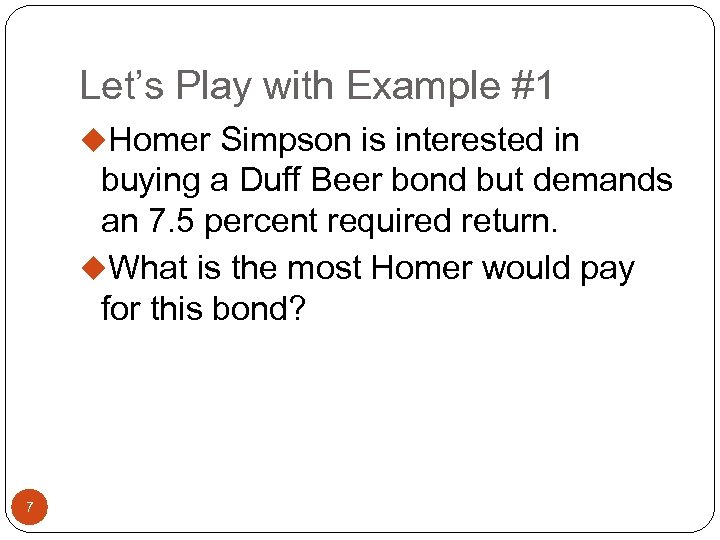Let’s Play with Example #1 u. Homer Simpson is interested in buying a Duff Beer bond but demands an 7. 5 percent required return. u. What is the most Homer would pay for this bond? 7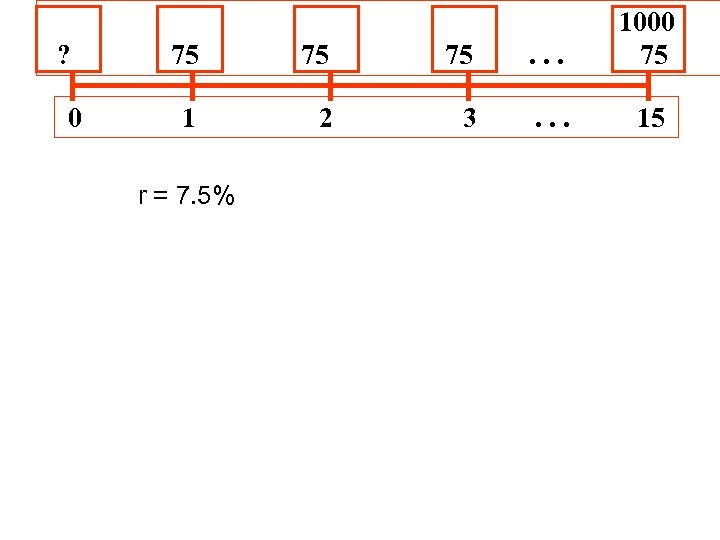? 0 75 75 75 . . . 1000 75 1 2 3 . . . 15 r = 7. 5%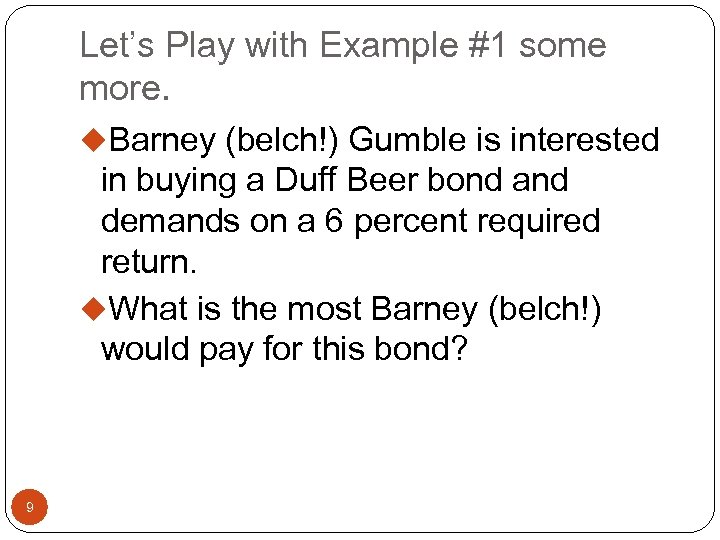Let’s Play with Example #1 some more. u. Barney (belch!) Gumble is interested in buying a Duff Beer bond and demands on a 6 percent required return. u. What is the most Barney (belch!) would pay for this bond? 9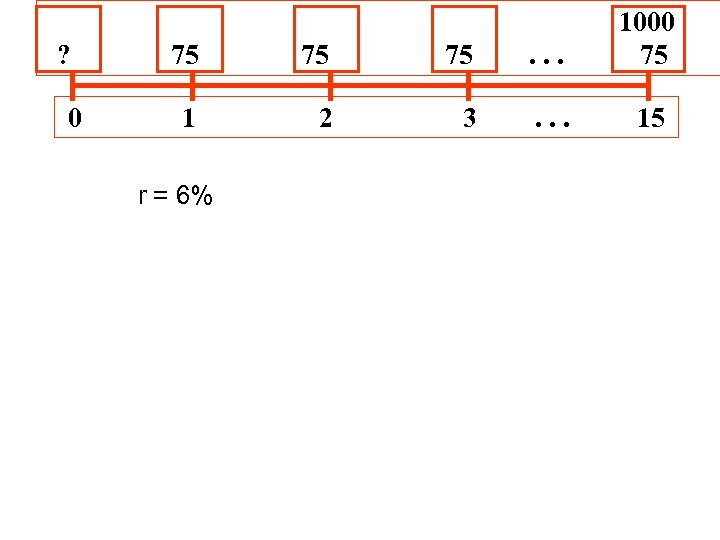? 0 75 75 75 . . . 1000 75 1 2 3 . . . 15 r = 6%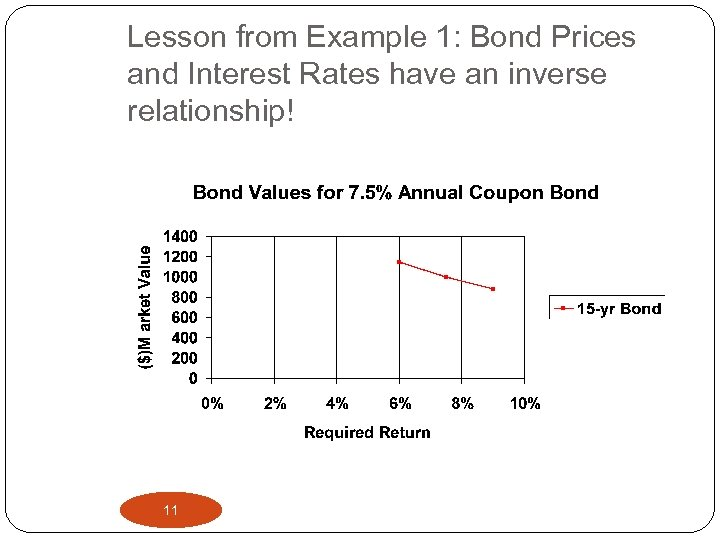Lesson from Example 1: Bond Prices and Interest Rates have an inverse relationship! 11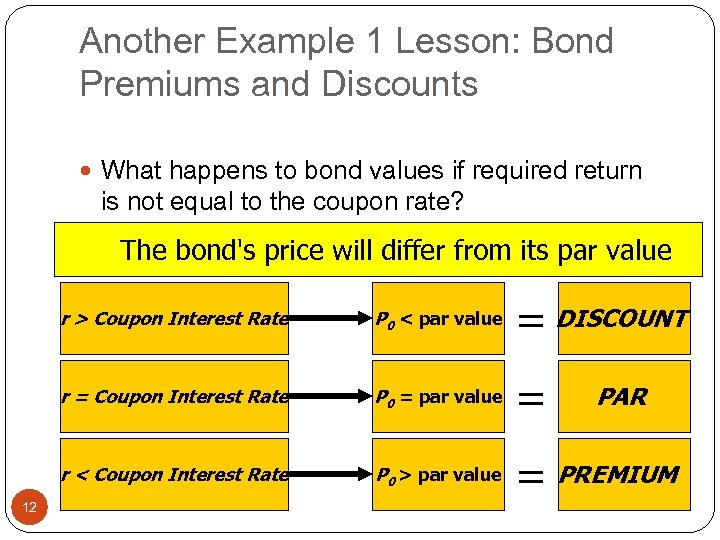Another Example 1 Lesson: Bond Premiums and Discounts What happens to bond values if required return is not equal to the coupon rate? The bond's price will differ from its par value r > Coupon Interest Rate = DISCOUNT r = Coupon Interest Rate P 0 = par value = r < Coupon Interest Rate 12 P 0 < par value P 0 > par value = PREMIUM PAR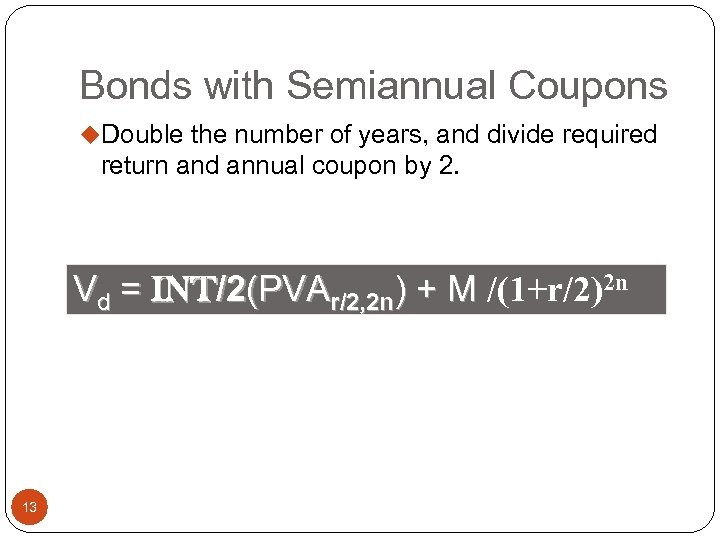Bonds with Semiannual Coupons u. Double the number of years, and divide required return and annual coupon by 2. Vd = INT/2(PVAr/2, 2 n) + M /(1+r/2)2 n 13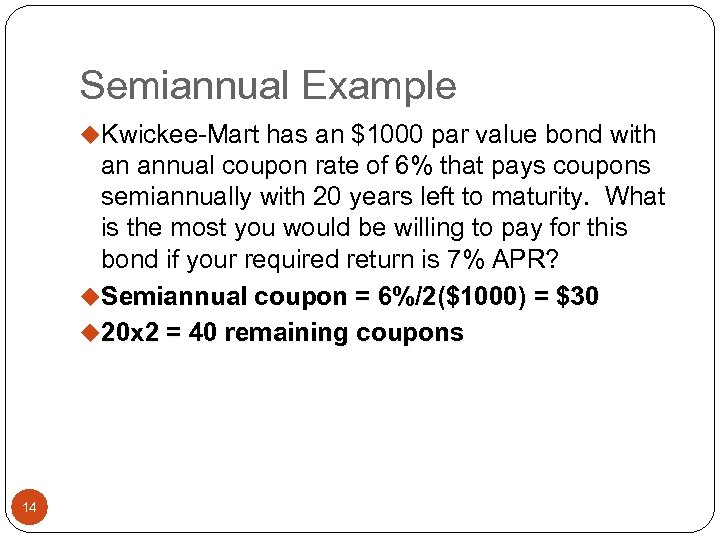Semiannual Example u. Kwickee-Mart has an \$1000 par value bond with an annual coupon rate of 6% that pays coupons semiannually with 20 years left to maturity. What is the most you would be willing to pay for this bond if your required return is 7% APR? u. Semiannual coupon = 6%/2(\$1000) = \$30 u 20 x 2 = 40 remaining coupons 14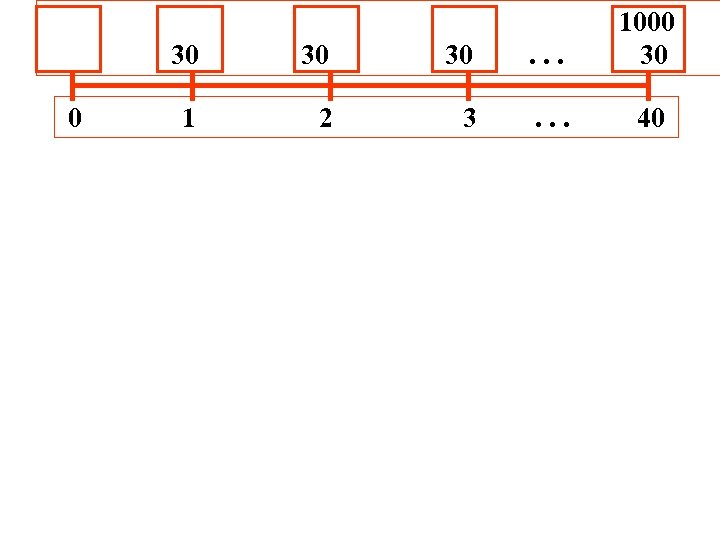30 0 30 30 . . . 1000 30 1 2 3 . . . 40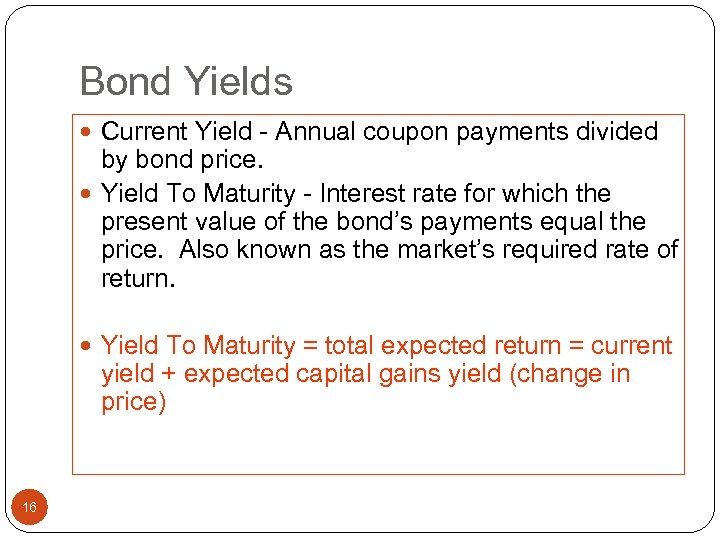Bond Yields Current Yield - Annual coupon payments divided by bond price. Yield To Maturity - Interest rate for which the present value of the bond’s payments equal the price. Also known as the market’s required rate of return. Yield To Maturity = total expected return = current yield + expected capital gains yield (change in price) 16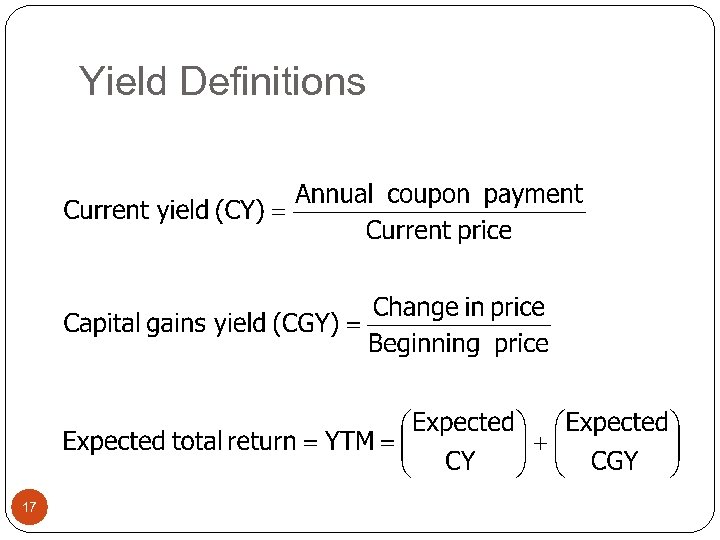Yield Definitions 17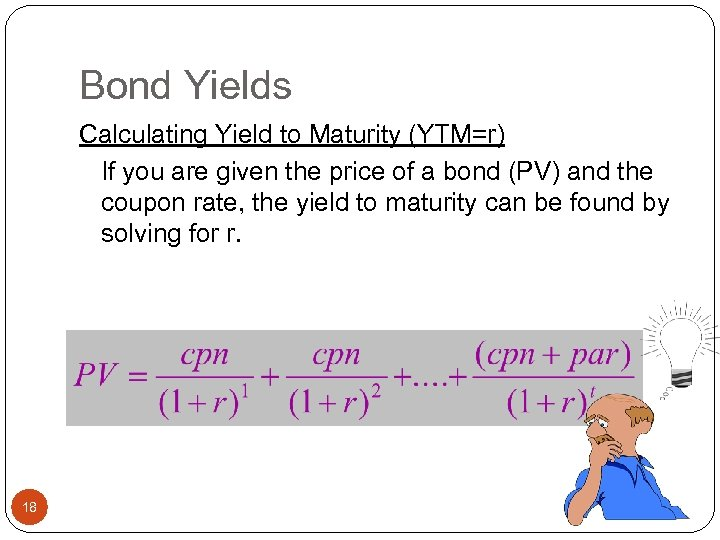Bond Yields Calculating Yield to Maturity (YTM=r) If you are given the price of a bond (PV) and the coupon rate, the yield to maturity can be found by solving for r. 18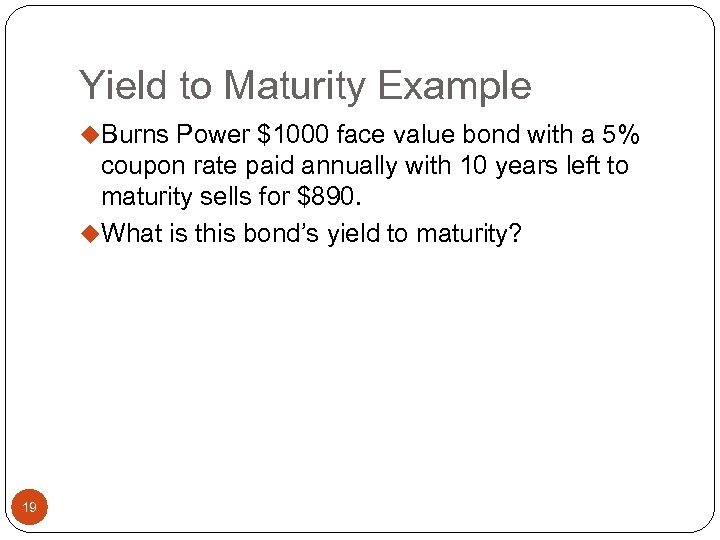Yield to Maturity Example u. Burns Power \$1000 face value bond with a 5% coupon rate paid annually with 10 years left to maturity sells for \$890. u. What is this bond’s yield to maturity? 19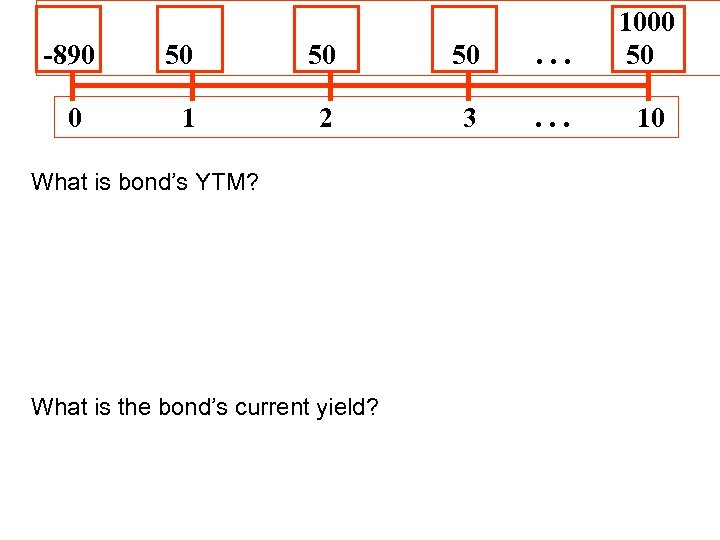-890 0 50 50 50 . . . 1000 50 1 2 3 . . . 10 What is bond’s YTM? What is the bond’s current yield?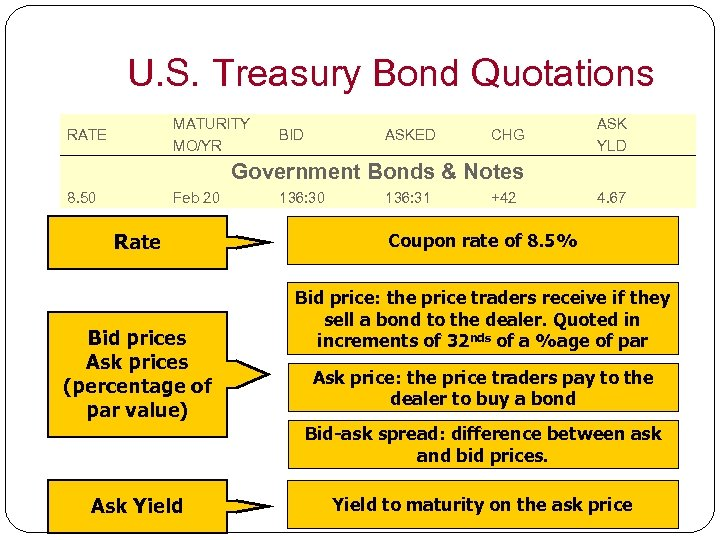U. S. Treasury Bond Quotations MATURITY MO/YR RATE BID ASKED CHG ASK YLD Government Bonds & Notes 8. 50 Feb 20 Rate Bid prices Ask prices (percentage of par value) 136: 30 136: 31 +42 4. 67 Coupon rate of 8. 5% Bid price: the price traders receive if they sell a bond to the dealer. Quoted in increments of 32 nds of a %age of par Ask price: the price traders pay to the dealer to buy a bond Bid-ask spread: difference between ask and bid prices. Ask Yield 21 Yield to maturity on the ask price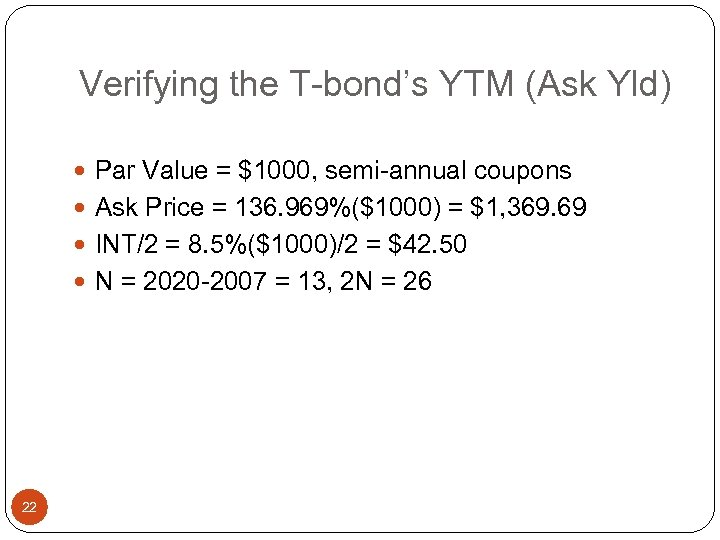Verifying the T-bond’s YTM (Ask Yld) Par Value = \$1000, semi-annual coupons Ask Price = 136. 969%(\$1000) = \$1, 369. 69 INT/2 = 8. 5%(\$1000)/2 = \$42. 50 N = 2020 -2007 = 13, 2 N = 26 22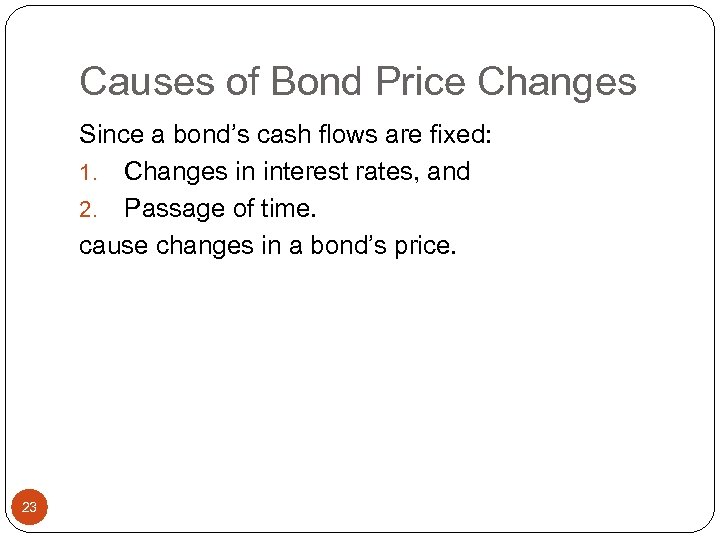Causes of Bond Price Changes Since a bond’s cash flows are fixed: 1. Changes in interest rates, and 2. Passage of time. cause changes in a bond’s price. 23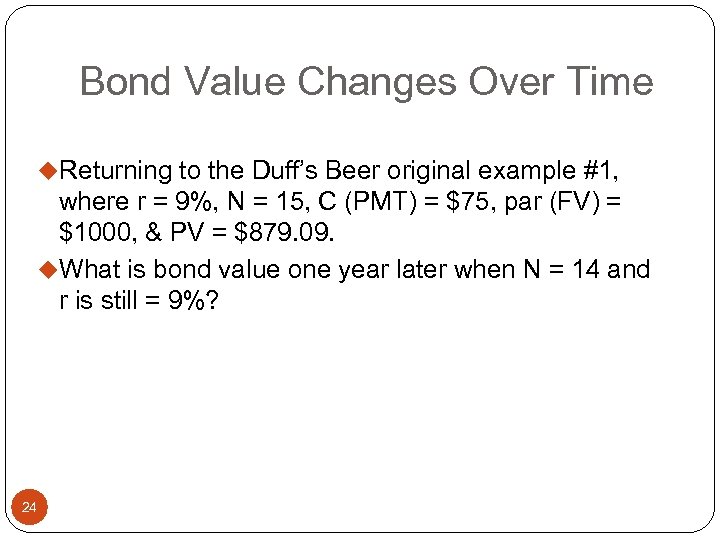Bond Value Changes Over Time u. Returning to the Duff’s Beer original example #1, where r = 9%, N = 15, C (PMT) = \$75, par (FV) = \$1000, & PV = \$879. 09. u. What is bond value one year later when N = 14 and r is still = 9%? 24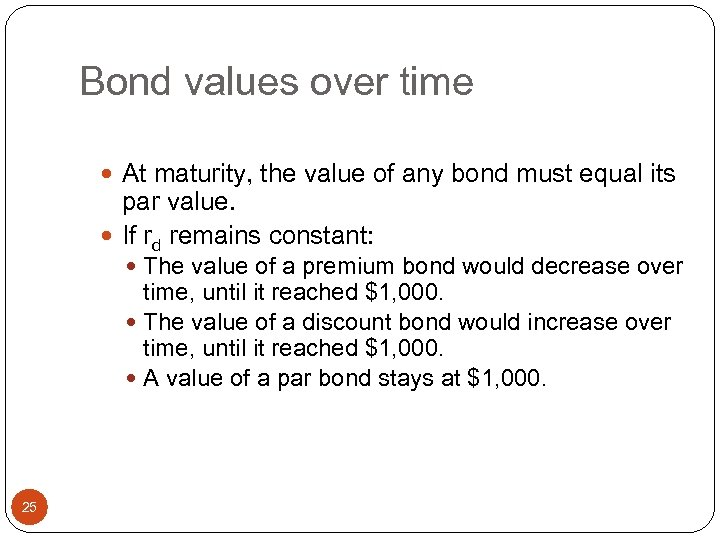Bond values over time At maturity, the value of any bond must equal its par value. If rd remains constant: The value of a premium bond would decrease over time, until it reached \$1, 000. The value of a discount bond would increase over time, until it reached \$1, 000. A value of a par bond stays at \$1, 000. 25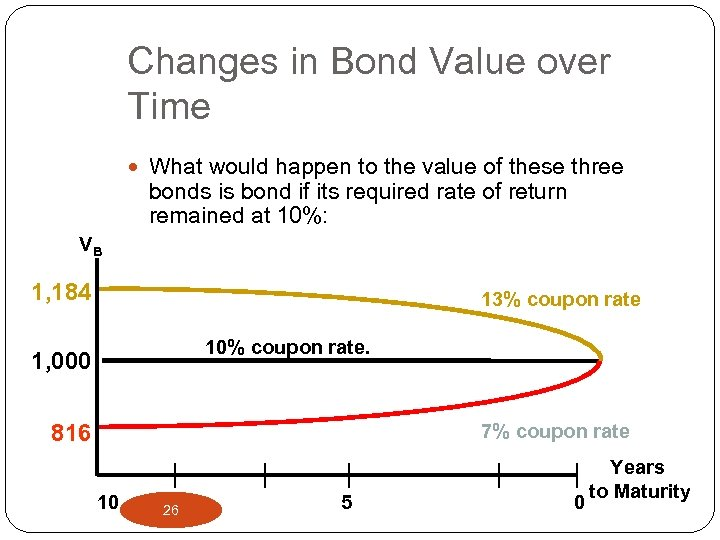Changes in Bond Value over Time What would happen to the value of these three bonds is bond if its required rate of return remained at 10%: VB 1, 184 13% coupon rate 10% coupon rate. 1, 000 816 7% coupon rate 10 26 5 0 Years to Maturity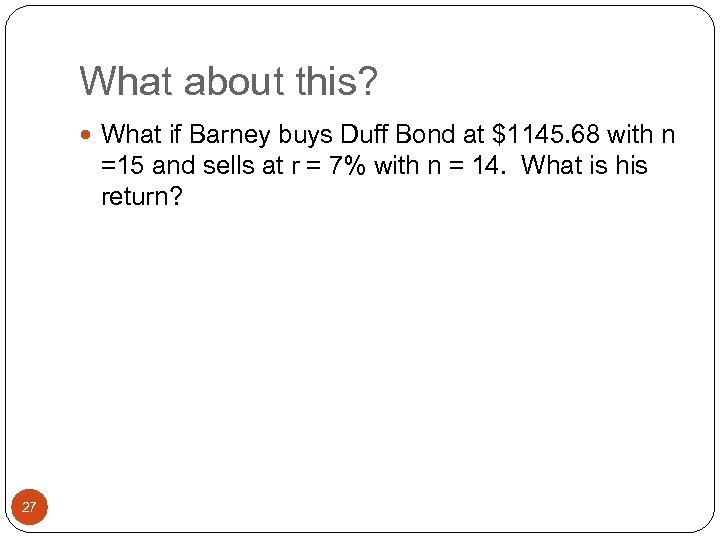What about this? What if Barney buys Duff Bond at \$1145. 68 with n =15 and sells at r = 7% with n = 14. What is his return? 27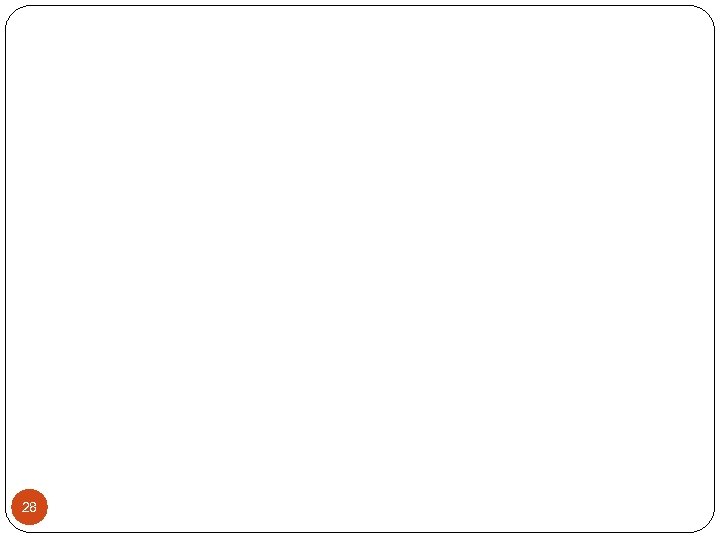28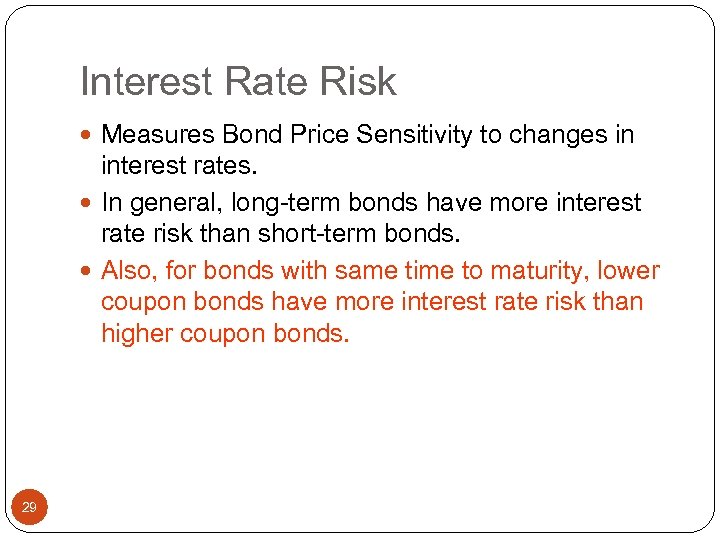Interest Rate Risk Measures Bond Price Sensitivity to changes in interest rates. In general, long-term bonds have more interest rate risk than short-term bonds. Also, for bonds with same time to maturity, lower coupon bonds have more interest rate risk than higher coupon bonds. 29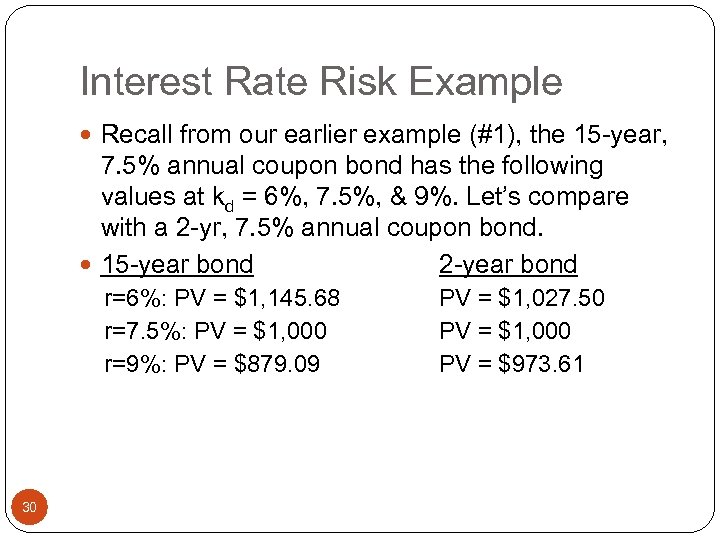Interest Rate Risk Example Recall from our earlier example (#1), the 15 -year, 7. 5% annual coupon bond has the following values at kd = 6%, 7. 5%, & 9%. Let’s compare with a 2 -yr, 7. 5% annual coupon bond. 15 -year bond 2 -year bond r=6%: PV = \$1, 145. 68 r=7. 5%: PV = \$1, 000 r=9%: PV = \$879. 09 30 PV = \$1, 027. 50 PV = \$1, 000 PV = \$973. 61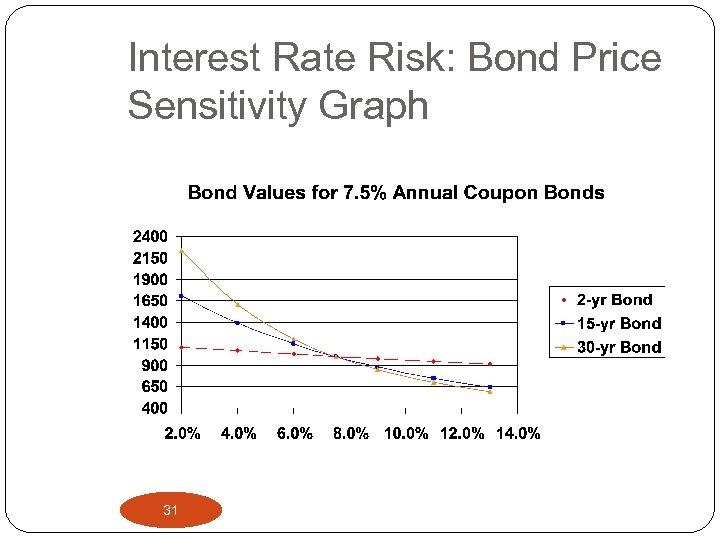Interest Rate Risk: Bond Price Sensitivity Graph 31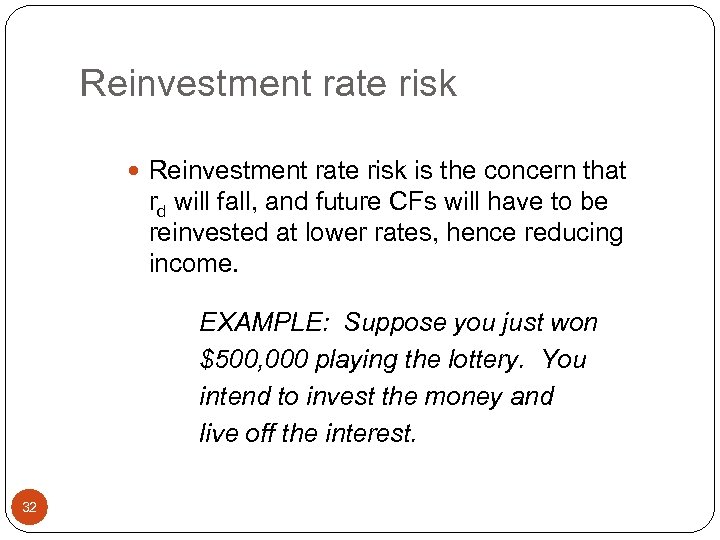Reinvestment rate risk is the concern that rd will fall, and future CFs will have to be reinvested at lower rates, hence reducing income. EXAMPLE: Suppose you just won \$500, 000 playing the lottery. You intend to invest the money and live off the interest. 32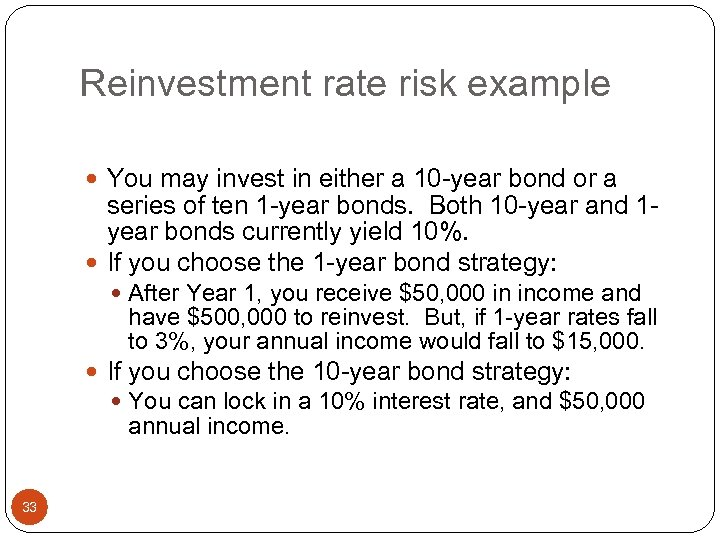Reinvestment rate risk example You may invest in either a 10 -year bond or a series of ten 1 -year bonds. Both 10 -year and 1 year bonds currently yield 10%. If you choose the 1 -year bond strategy: After Year 1, you receive \$50, 000 in income and have \$500, 000 to reinvest. But, if 1 -year rates fall to 3%, your annual income would fall to \$15, 000. If you choose the 10 -year bond strategy: You can lock in a 10% interest rate, and \$50, 000 annual income. 33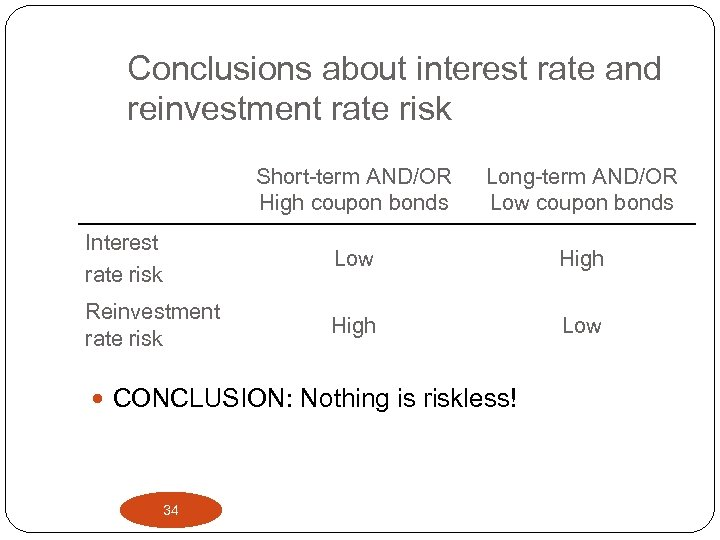Conclusions about interest rate and reinvestment rate risk Short-term AND/OR High coupon bonds Long-term AND/OR Low coupon bonds Interest rate risk Low High Reinvestment rate risk High Low CONCLUSION: Nothing is riskless! 34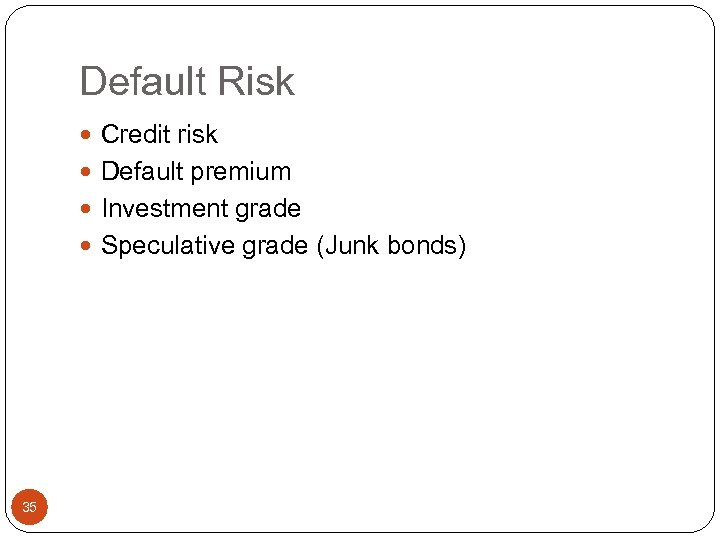Default Risk Credit risk Default premium Investment grade Speculative grade (Junk bonds) 35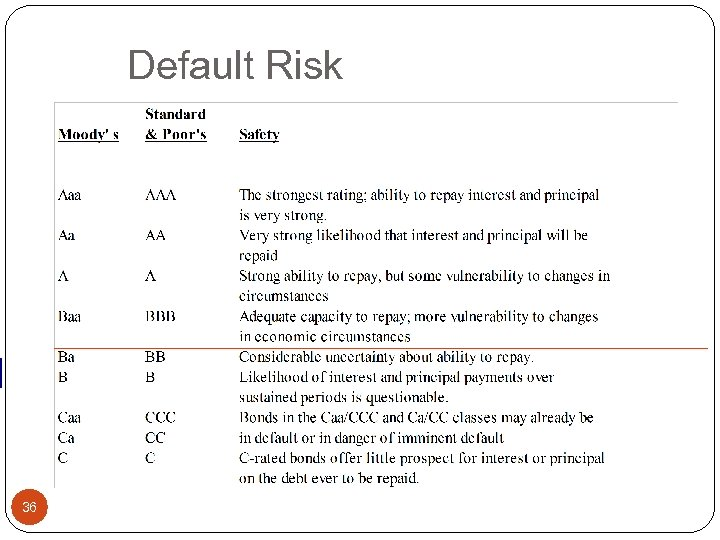Default Risk 36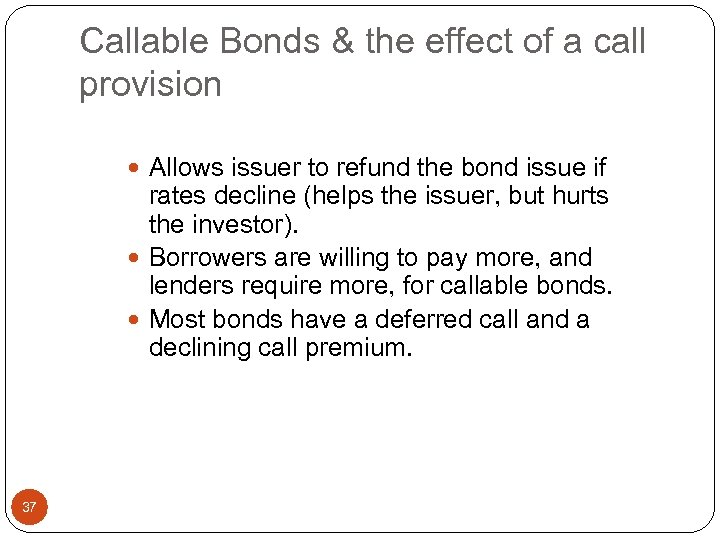Callable Bonds & the effect of a call provision Allows issuer to refund the bond issue if rates decline (helps the issuer, but hurts the investor). Borrowers are willing to pay more, and lenders require more, for callable bonds. Most bonds have a deferred call and a declining call premium. 37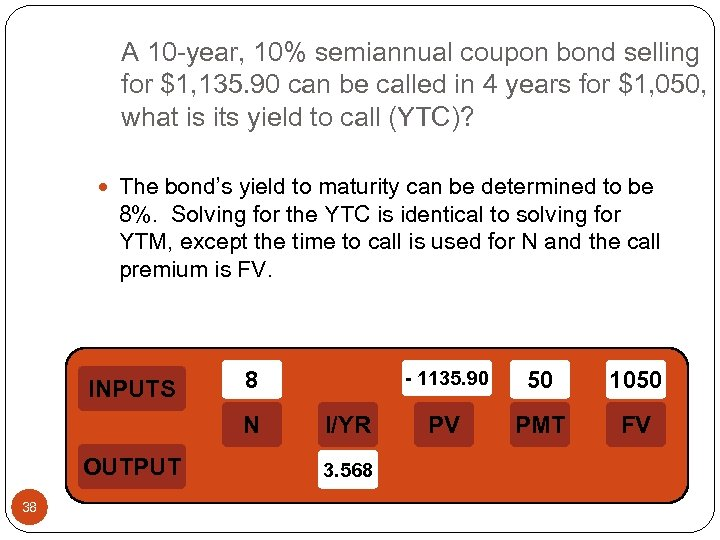A 10 -year, 10% semiannual coupon bond selling for \$1, 135. 90 can be called in 4 years for \$1, 050, what is its yield to call (YTC)? The bond’s yield to maturity can be determined to be 8%. Solving for the YTC is identical to solving for YTM, except the time to call is used for N and the call premium is FV. INPUTS 8 N OUTPUT 38 - 1135. 90 I/YR 3. 568 50 1050 PV PMT FV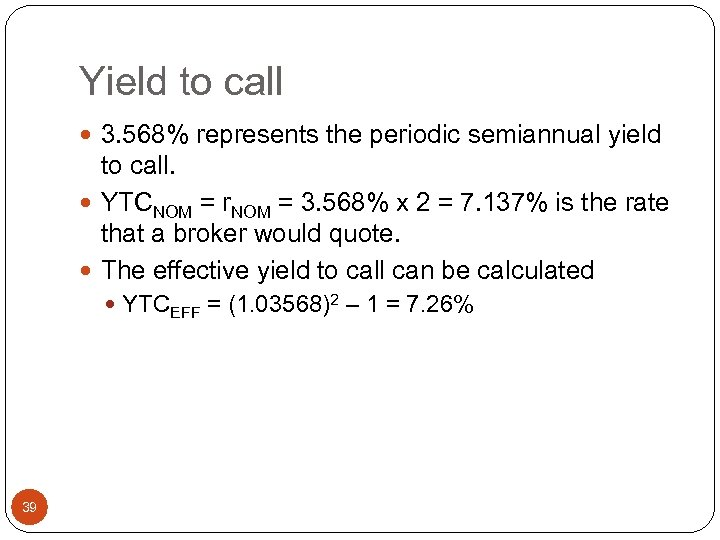Yield to call 3. 568% represents the periodic semiannual yield to call. YTCNOM = r. NOM = 3. 568% x 2 = 7. 137% is the rate that a broker would quote. The effective yield to call can be calculated YTCEFF = (1. 03568)2 – 1 = 7. 26% 39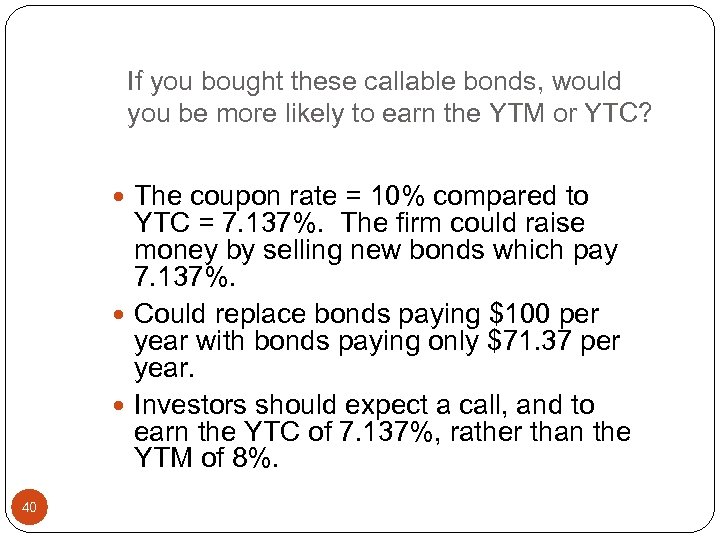If you bought these callable bonds, would you be more likely to earn the YTM or YTC? The coupon rate = 10% compared to YTC = 7. 137%. The firm could raise money by selling new bonds which pay 7. 137%. Could replace bonds paying \$100 per year with bonds paying only \$71. 37 per year. Investors should expect a call, and to earn the YTC of 7. 137%, rather than the YTM of 8%. 40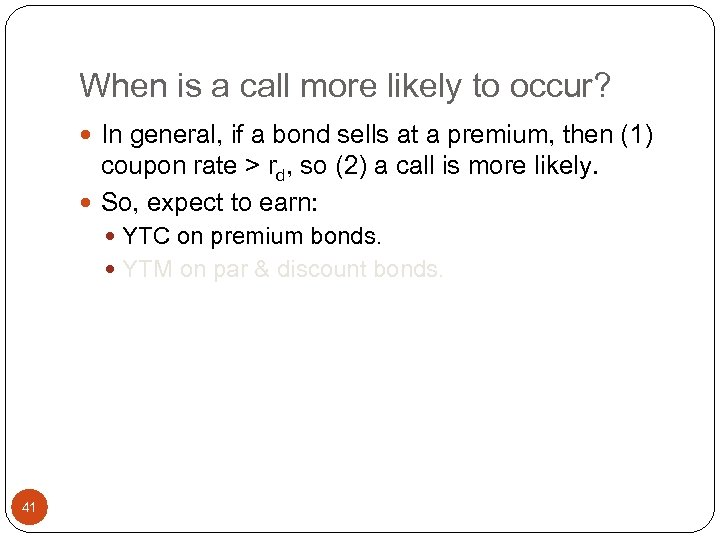When is a call more likely to occur? In general, if a bond sells at a premium, then (1) coupon rate > rd, so (2) a call is more likely. So, expect to earn: YTC on premium bonds. YTM on par & discount bonds. 41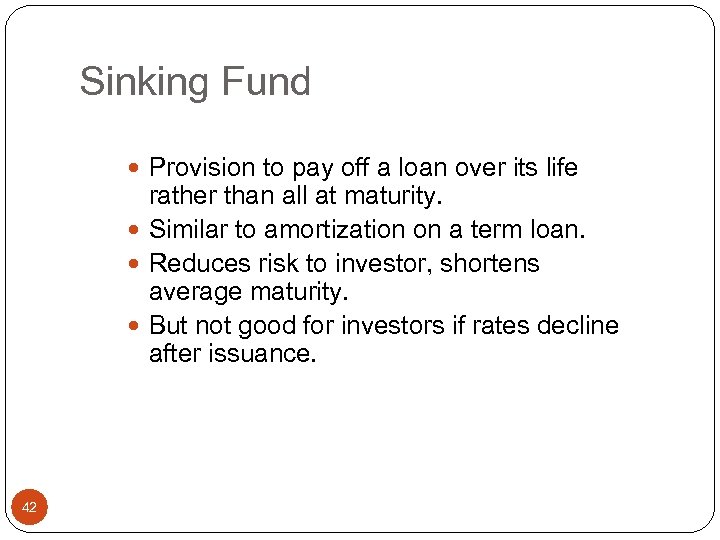Sinking Fund Provision to pay off a loan over its life rather than all at maturity. Similar to amortization on a term loan. Reduces risk to investor, shortens average maturity. But not good for investors if rates decline after issuance. 42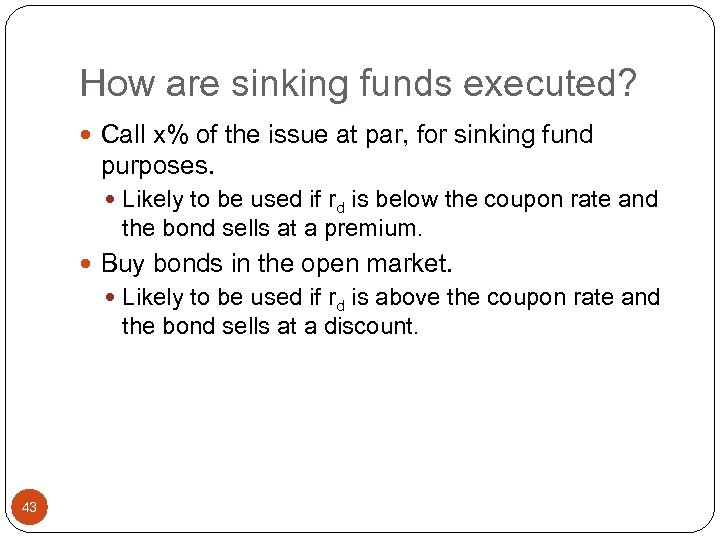How are sinking funds executed? Call x% of the issue at par, for sinking fund purposes. Likely to be used if rd is below the coupon rate and the bond sells at a premium. Buy bonds in the open market. Likely to be used if rd is above the coupon rate and the bond sells at a discount. 43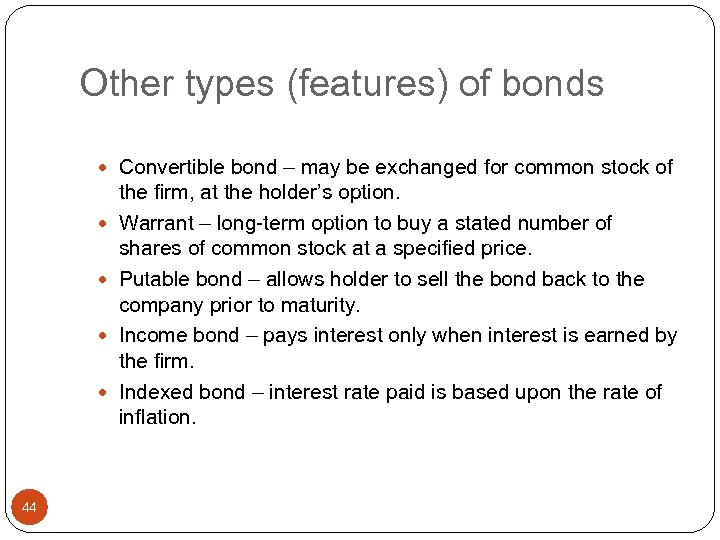Other types (features) of bonds Convertible bond – may be exchanged for common stock of 44 the firm, at the holder’s option. Warrant – long-term option to buy a stated number of shares of common stock at a specified price. Putable bond – allows holder to sell the bond back to the company prior to maturity. Income bond – pays interest only when interest is earned by the firm. Indexed bond – interest rate paid is based upon the rate of inflation.